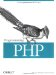4.6 Comparing Strings

PHP has two operators and six functions for comparing strings to each other.

4.6.1 Exact Comparisons

You can compare two strings for equality with the == and === operators. These operators differ in how they deal with non-string operands. The == operator casts non-string operands to strings, so it reports that 3 and "3" are equal. The === operator does not cast, and returns false if the types of the arguments differ.

\$o1 = 3; \$o2 = "3"; if (\$o1 == \$o2) {   echo("== returns true<br>"); } if (\$o1 === \$o2) {   echo("=== returns true<br>"); } == returns true

The comparison operators (<, <=, >, >=) also work on strings:

\$him = "Fred"; \$her = "Wilma"; if (\$him < \$her) {   print "\$him comes before \$her in the alphabet.\n"; } Fred comes before Wilma in the alphabet

However, the comparison operators give unexpected results when comparing strings and numbers:

\$string = "PHP Rocks"; \$number = 5; if (\$string < \$number) {   echo("\$string < \$number"); } PHP Rocks < 5

When one argument to a comparison operator is a number, the other argument is cast to a number. This means that "PHP Rocks" is cast to a number, giving 0 (since the string does not start with a number). Because 0 is less than 5, PHP prints "PHP Rocks < 5".

To explicitly compare two strings as strings, casting numbers to strings if necessary, use the strcmp( ) function:

\$relationship = strcmp(string_1, string_2);

The function returns a number less than 0 if string_1 sorts before string_2, greater than 0 if string_2 sorts before string_1, or 0 if they are the same:

\$n = strcmp("PHP Rocks", 5); echo(\$n);                              1

A variation on strcmp( ) is strcasecmp( ) , which converts strings to lowercase before comparing them. Its arguments and return values are the same as those for strcmp( ):

\$n = strcasecmp("Fred", "frED");       // \$n is 0

Another variation on string comparison is to compare only the first few characters of the string. The strncmp( ) and strncasecmp( ) functions take an additional argument, the initial number of characters to use for the comparisons:

\$relationship = strncmp(string_1, string_2, len); \$relationship = strncasecmp(string_1, string_2, len);

The final variation on these functions is natural-order comparison with strnatcmp( ) and strnatcasecmp( ), which take the same arguments as strcmp( ) and return the same kinds of values. Natural-order comparison identifies numeric portions of the strings being compared and sorts the string parts separately from the numeric parts.

Table 4-5 shows strings in natural order and ASCII order.

Natural order

ASCII order

pic1.jpg
pic1.jpg
pic5.jpg
pic10.jpg
pig10.jpg
pic5.jpg
pic50.jpg
pic50.jpg

4.6.2 Approximate Equality

PHP provides several functions that let you test whether two strings are approximately equal: soundex( ) , metaphone( ), similar_text(), and levenshtein( ).

\$soundex_code = soundex(\$string); \$metaphone_code = metaphone(\$string); \$in_common = similar_text(\$string_1, \$string_2 [, \$percentage ]); \$similarity = levenshtein(\$string_1, \$string_2); \$similarity = levenshtein(\$string_1, \$string_2 [, \$cost_ins, \$cost_rep, \$cost_del ]);

The Soundex and Metaphone algorithms each yield a string that represents roughly how a word is pronounced in English. To see whether two strings are approximately equal with these algorithms, compare their pronunciations. You can compare Soundex values only to Soundex values and Metaphone values only to Metaphone values. The Metaphone algorithm is generally more accurate, as the following example demonstrates:

\$known = "Fred"; \$query = "Phred"; if (soundex(\$known) == soundex(\$query)) {   print "soundex: \$known sounds \$query<br>"; } else {   print "soundex: \$known doesn't sound like \$query<br>"; } if (metaphone(\$known) == metaphone(\$query)) {   print "metaphone: \$known sounds \$query<br>"; } else {   print "metaphone: \$known doesn't sound like \$query<br>"; } soundex: Fred doesn't sound like Phred metaphone: Fred sounds like Phred

The similar_text( ) function returns the number of characters that its two string arguments have in common. The third argument, if present, is a variable in which to store the commonality as a percentage:

\$string_1 = "Rasmus Lerdorf"; \$string_2 = "Razmus Lehrdorf"; \$common = similar_text(\$string_1, \$string_2, \$percent); printf("They have %d chars in common (%.2f%%).", \$common, \$percent); They have 13 chars in common (89.66%).

The Levenshtein algorithm calculates the similarity of two strings based on how many characters you must add, substitute, or remove to make them the same. For instance, "cat" and "cot" have a Levenshtein distance of 1, because you need to change only one character (the "a" to an "o") to make them the same:

\$similarity = levenshtein("cat", "cot");         // \$similarity is 1

This measure of similarity is generally quicker to calculate than that used by the similar_text( ) function. Optionally, you can pass three values to the levenshtein( ) function to individually weight insertions, deletions, and replacements for instance, to compare a word against a contraction.

This example excessively weights insertions when comparing a string against its possible contraction, because contractions should never insert characters:

echo levenshtein('would not', 'wouldn\'t', 500, 1, 1);Programming PHP
ISBN: 1565926102
EAN: 2147483647
Year: 2007
Pages: 168

Similar book on Amazon